HOTS Questions: Motion

# HOTS Questions: Motion

Test Description

## 25 Questions MCQ Test General Science(Prelims) by IRS Divey Sethi | HOTS Questions: Motion

HOTS Questions: Motion for Class 9 2022 is part of General Science(Prelims) by IRS Divey Sethi preparation. The HOTS Questions: Motion questions and answers have been prepared according to the Class 9 exam syllabus.The HOTS Questions: Motion MCQs are made for Class 9 2022 Exam. Find important definitions, questions, notes, meanings, examples, exercises, MCQs and online tests for HOTS Questions: Motion below.
Solutions of HOTS Questions: Motion questions in English are available as part of our General Science(Prelims) by IRS Divey Sethi for Class 9 & HOTS Questions: Motion solutions in Hindi for General Science(Prelims) by IRS Divey Sethi course. Download more important topics, notes, lectures and mock test series for Class 9 Exam by signing up for free. Attempt HOTS Questions: Motion | 25 questions in 25 minutes | Mock test for Class 9 preparation | Free important questions MCQ to study General Science(Prelims) by IRS Divey Sethi for Class 9 Exam | Download free PDF with solutions
 1 Crore+ students have signed up on EduRev. Have you?
HOTS Questions: Motion - Question 1

### A body moves in a uniform circular motion

Detailed Solution for HOTS Questions: Motion - Question 1 Accelerating objects are objects which are changing their velocity - either the speed (i.e., magnitude of the velocity vector) or the direction. An object undergoing uniform circular motion is moving with a constant speed. Nonetheless, it is accelerating due to its change in direction.
HOTS Questions: Motion - Question 2

### 180° plane angle is equal to

Detailed Solution for HOTS Questions: Motion - Question 2

π radian is equal to 180 ° . Radian is a unit of measurement for angles, just like degrees are, and π is just the no. of radians that makes up that angle.

HOTS Questions: Motion - Question 3

### Speed of a body in particular direction can be called

Detailed Solution for HOTS Questions: Motion - Question 3 Distance covered by a body in per unit time is called Speed & Distance covered by a body in a given direction is known as Velocity ....... It can also be written as speed of a body in a particular direction is called Velocity .
HOTS Questions: Motion - Question 4

Statement A: Uniform circular motion is a case of accelerated motion
Statement B: In third equation of motion we do not have the time.

Detailed Solution for HOTS Questions: Motion - Question 4

Uniform circular motion is always accelerated because the direction of velocity is continuously changing hence velocity is changing which causes change in acceleration. Hence the given statement is true.
There are 3 equations of motion,

• v = u+ at
• s = ut + 1 /2 at
• v2 = u2 + 2as

As, we can see there , we don't have the time in the third equation of motion.

HOTS Questions: Motion - Question 5

Which of the following is correct about uniform circular motion?
A. Direction of motion is continuously changed
B. Direction of motion is not changed
C. Speed and direction both remain constant
D. Speed is constant, but direction is changing

Detailed Solution for HOTS Questions: Motion - Question 5

Uniform circular motion is accelerated because the velocity changes due to continuous change in the direction of motion. So, even when the body moves ta a constant speed, its velocity is not constant. Thus, for a body moving in a circular path at a constant speed, its velocity changes continuously. The change in velocity gives rise to an acceleration in the moving body. Therefore circular motion is an acceleration motion even when the speed remains constant.

HOTS Questions: Motion - Question 6

Which of the quantities – speed, time, velocity and acceleration have the same SI unit?

HOTS Questions: Motion - Question 7

Find the correct statement

Detailed Solution for HOTS Questions: Motion - Question 7

When the velocity decreases with respect to time it is termed as retardation or deceleration. In reality negative acceleration is commonly called retardation. Its SI unit. is m/s2.

HOTS Questions: Motion - Question 8

A body is said to be in rest when

Detailed Solution for HOTS Questions: Motion - Question 8  If philosophically speaking one could be physically at rest but mentally in motion for example when one is taking a nap, except one is dead, one's internal system is constantly in motion as breathing occurs, dreams, digestion etc. Rest is a state in physics that refers to an object being stationary relative to a particular frame of reference or another object; when the position of a body with respect to its surroundings does not change with time it is said to be "at rest".
HOTS Questions: Motion - Question 9

A bus moves from stop ‘A to stop ‘B’ with a speed of 40 km/hr and then from stop ‘B’ to stop ‘A with a speed of 50 km/hr. Its average speed is

Detailed Solution for HOTS Questions: Motion - Question 9

Let the distance between A and B be D and time taken to cover the distance be T1 and T2.

Speed = Distance/Time

40 = (D/T1) and 50 = (D/T2)

T1 = (D/40)

also, T2 = (D/50)

Total time (T) = T1 + T2

⇒ (D/40) + (D/50) = (9D/200)

Total Distance = (D + D) = 2D

Average speed = Total Distance/Total Time Taken

= (2D/(9D/200)) = 400/9 = 44.4 km/hr

HOTS Questions: Motion - Question 10

Find the incorrect statement

Detailed Solution for HOTS Questions: Motion - Question 10

When a body moves with constant speed its acceleration is zero . The body is said to be accelerating if it moves in a uniform circular motion. The slope of the velocity- time graph gives Instantaneous acceleration . All the given statements are correct. Therefore ,  option ( d) is correct answer.

HOTS Questions: Motion - Question 11

Match the following with correct response.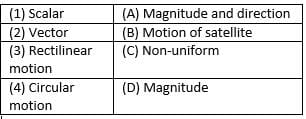HOTS Questions: Motion - Question 12

Match the following with correct response.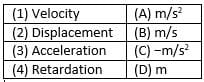HOTS Questions: Motion - Question 13

Match the following with correct response.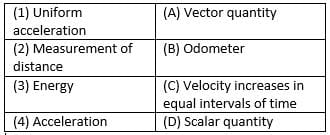Detailed Solution for HOTS Questions: Motion - Question 13

Vector- A physical quantity having a magnitude as well as direction is known as vector quantity.
Odometer - an instrument for measuring the distance travelled by a wheeled vehicle.
Acceleration of a body is defined as the rate of change of its velocity with time.
Scalar - A physical quantity having only magnitude is known as a scalar quantity.

HOTS Questions: Motion - Question 14

The area under the velocity – time graph gives the

Detailed Solution for HOTS Questions: Motion - Question 14

The correct answer is B as velocity-time graph we will get displacement travelled.as ,displacement=velocity/time

HOTS Questions: Motion - Question 15

Which of the following is a false statement

Detailed Solution for HOTS Questions: Motion - Question 15 A body can have a constant speed but a changing velocity because the direction can change while the speed is constant. (A car goes 50 mph around a roundabout). However, a body can not have a constant velocity with a changing speed.
HOTS Questions: Motion - Question 16

Is the following graph possible ?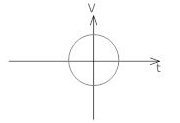Detailed Solution for HOTS Questions: Motion - Question 16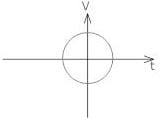The graph shown above is not possible in case of Velocity - time graph. Graph may be straight  curved but not completely circular.

HOTS Questions: Motion - Question 17

Which of the following situations are not possible?

Detailed Solution for HOTS Questions: Motion - Question 17

Yes, a body can have a constant speed and still be accelerating.
Acceleration can be either due to change in speed or due to direction of motion or both.
Consider an example of a uniform circular motion. In a uniform circular motion, the body moves with a constant speed but we still say that it is accelerating due to the change in direction
This acceleration is called centripetal acceleration and is given as
a​= −v2/r​
where v= constant speed

HOTS Questions: Motion - Question 18

If we get a curve line in displacement – time graph then the velocity is

HOTS Questions: Motion - Question 19

Match the following with correct response.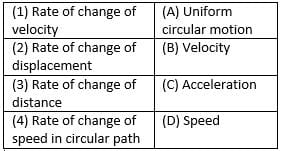HOTS Questions: Motion - Question 20

Match the following with correct response.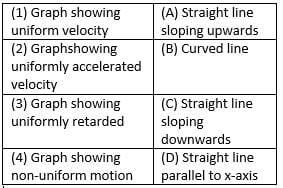HOTS Questions: Motion - Question 21

Which of the following statement is correct?
A. When a body starts from rest, u = 0
B. When a body comes to rest, V = 0
C. For a body under free fall, a = −9.8m/s2
D. For uniform velocity, a = 0

Detailed Solution for HOTS Questions: Motion - Question 21

Acceleration is positive in the “down” direction, where “down” is where the nearest big source of gravity is.

In physics, acceleration is usually expressed as a vector, i.e. a direction and a magnitude. A magnitude is an absolute value, hence always positive.

HOTS Questions: Motion - Question 22

The slope of distance – time graph gives instantaneous.

Detailed Solution for HOTS Questions: Motion - Question 22 The gradient of a distance-time graph represents the speed of an object. The velocity of an object is its speed in a particular direction. The slope on a velocity-time graph represents the acceleration of an object. The distance travelled is equal to the area under a velocity-time graph.
HOTS Questions: Motion - Question 23

Distance is a __________ quantity

Detailed Solution for HOTS Questions: Motion - Question 23

Distance is a scalar quantity that refers to "how much ground an object has covered" during its motion.

HOTS Questions: Motion - Question 24

If a body doesn't rotates then its motion is

Detailed Solution for HOTS Questions: Motion - Question 24 The correct answer is B because this applies in the case of inertia. if you are travelling on a vehicle you are not doing and movement but the vehicle is moving then at that time you are at rest as well as at motion.
HOTS Questions: Motion - Question 25

A body is moving with a velocity of 10m/s. If the motion is uniform, what will be the velocity after 10 second?

Detailed Solution for HOTS Questions: Motion - Question 25

As the body is moving in uniform motion, so the acceleration will be zero.
as acceleration is zero, the change in velocity is also zero.it means velocity will be the same in whole motion.

## General Science(Prelims) by IRS Divey Sethi

39 videos|110 docs|255 tests
 Use Code STAYHOME200 and get INR 200 additional OFF Use Coupon Code
Information about HOTS Questions: Motion Page
In this test you can find the Exam questions for HOTS Questions: Motion solved & explained in the simplest way possible. Besides giving Questions and answers for HOTS Questions: Motion, EduRev gives you an ample number of Online tests for practice

## General Science(Prelims) by IRS Divey Sethi

39 videos|110 docs|255 tests